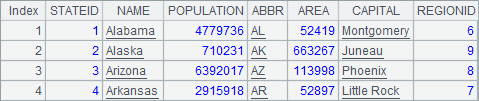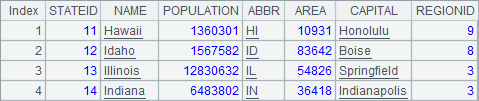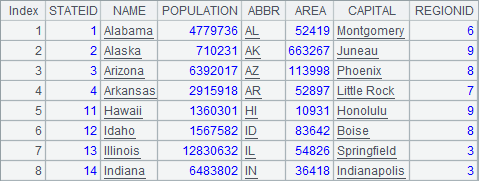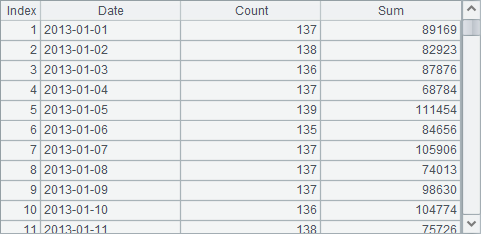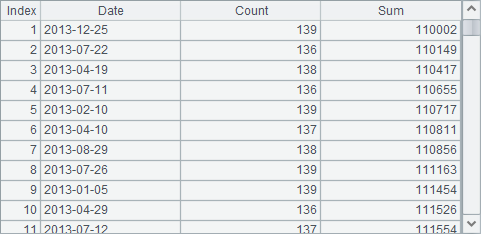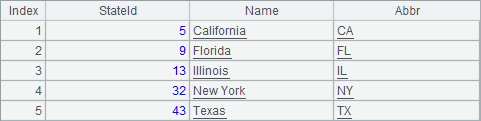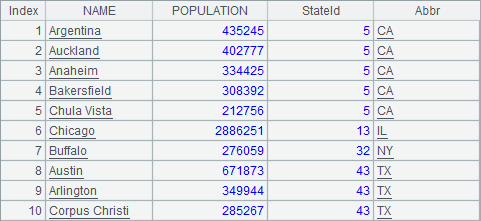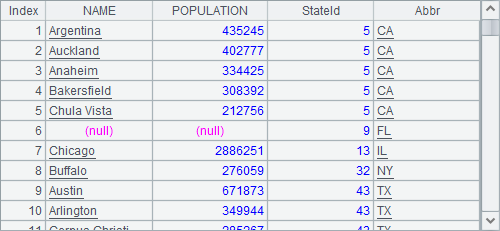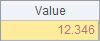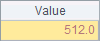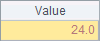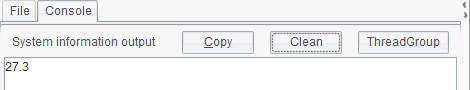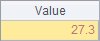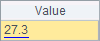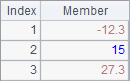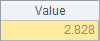# 图元的基本属性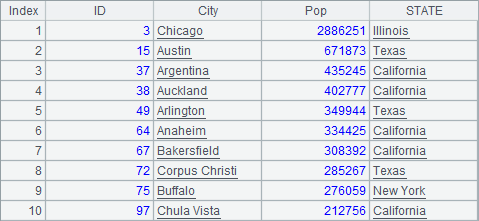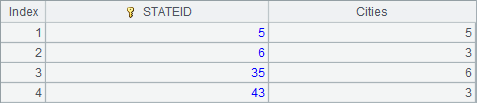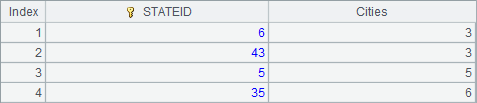A 1 =canvas() 2 =demo.query("select * from GYMSCORE where EVENT = 'Vault'") 3 =A1.plot("BackGround") 4 =A1.plot("NumericAxis","name":"y","location":2,"axisColor":-16776961, "axisLineStyle":2,"axisLineWeight":2,"labelFont":"Calibri", "labelOverlapping":true) 5 =A1.plot("EnumAxis","name":"x","categories":A2.(NAME), "labelFont":"Arial") 6 =A1.plot("Column","axis1":"x","data1":A2.(NAME),"axis2":"y", "data2":A2.(SCORE),"text":A2.(SCORE)) 7 =A1.draw@p(450,350)